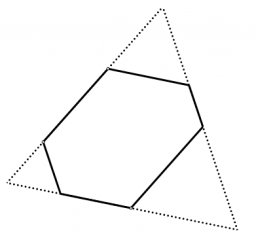# Irregular hexagon

There is an irregular hexagon whose sides are the same length. The opposite sides are parallel; their distance is 237, 195, and193. What is its area?

S =  89590.8868

### Step-by-step explanation:Did you find an error or inaccuracy? Feel free to write us. Thank you!

Showing 1 comment:
Math student
There is an irregular hexagon whose sides are the same length.
If  a1=a2=a3=a4=a5=a6 it is regular hexagon.
There is an irregular hexagon whose opposite sides are the same length....
Then is a=87,18... b=135,68... c=137,99... d=360,84...
Area of hexagons is:
S=Sd-Sa-Sb-Sc  =36875,1 (Sd; Sa; Sb; Sc is area of  equilateral triangels).

Tips for related online calculators
Need help calculating sum, simplifying, or multiplying fractions? Try our fraction calculator.